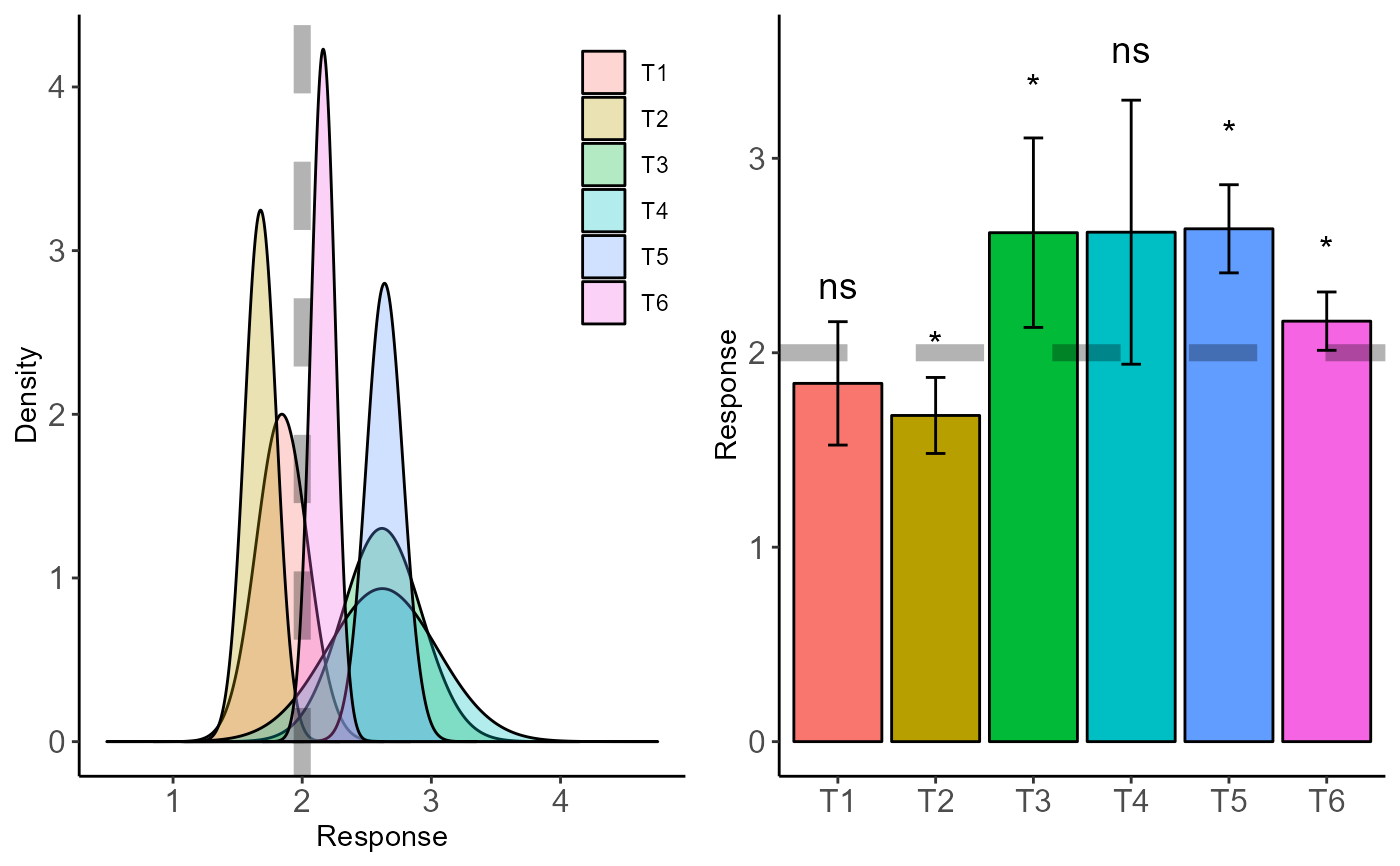Sometimes the researcher wants to test whether the treatment mean is greater than/equal to or less than a reference value. For example, I want to know if the average productivity of my treatment is higher than the average productivity of a given country. For this, this function allows comparing the means with a reference value using the t test.

plot_tonetest(tonetest, alpha = 0.95)

## Arguments

tonetest

t.one.test object

alpha

confidence level.

## Value

returns a density plot and a column plot to compare a reference value with other treatments.

## Author

Gabriel Danilo Shimizu

## Examples

library(AgroR)
data("pomegranate")
resu=tonetest(resp=pomegranate$WL, trat=pomegranate$trat, mu=2)
plot_tonetest(resu)